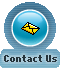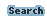Conditional Expressions Previous  Top  Next

Absolute Database provides the following functions to convert data from one data type to another:

 Function Description CASE Evaluates a list of conditions and returns one of multiple possible result expressions COALESCE Returns the first non-NULL expression from a list

CASE

Evaluates a list of conditions and returns one of multiple possible result expressions.
CASE has two formats:
 · The simple CASE function compares an expression to a set of simple expressions to determine the result.
 · The searched CASE function evaluates a set of Boolean expressions to determine the result.
Both formats support an optional ELSE argument.

Simple CASE function:

CASE input_expression
WHEN when_expression THEN result_expression
[ ...n ]
[
ELSE else_result_expression
]
END

Searched CASE function:

CASE
WHEN Boolean_expression THEN result_expression
[ ...n ]
[
ELSE else_result_expression
]
END

input_expression
Is the expression evaluated when using the simple CASE format. input_expression is any valid expression.

WHEN when_expression
Is a simple expression to which input_expression is compared when using the simple CASE format. when_expression is any valid expression. The data types of input_expression and each when_expression must be the same or must be an implicit conversion.

n
Is a placeholder indicating that multiple WHEN when_expression THEN result_expression clauses, or multiple WHEN Boolean_expression THEN result_expression clauses can be used.

THEN result_expression
Is the expression returned when input_expression equals when_expression evaluates to TRUE, or Boolean_expression evaluates to TRUE. result expression is any valid expression.

ELSE else_result_expression
Is the expression returned if no comparison operation evaluates to TRUE. If this argument is omitted and no comparison operation evaluates to TRUE, CASE returns NULL. else_result_expression is any valid expression. The data types of else_result_expression and any result_expression must be the same or must be an implicit conversion.

WHEN Boolean_expression
Is the Boolean expression evaluated when using the searched CASE format. Boolean_expression is any valid Boolean expression.

Result Values

Simple CASE function:

 · Evaluates input_expression, and then, in the order specified, evaluates input_expression = when_expression for each WHEN clause.
 · Returns the result_expression of the first (input_expression = when_expression) that evaluates to TRUE.
 · If no input_expression = when_expression evaluates to TRUE, Absolute Database returns the else_result_expression if an ELSE clause is specified, or a NULL value if no ELSE clause is specified.

Searched CASE function:

 · Evaluates, in the order specified, Boolean_expression for each WHEN clause.
 · Returns result_expression of the first Boolean_expression that evaluates to TRUE.
 · If no Boolean_expression evaluates to TRUE, Absolute Database returns the else_result_expression if an ELSE clause is specified, or a NULL value if no ELSE clause is specified.

Examples:

SELECT first_name, last_name,
CASE state
WHEN 'CA' THEN 'California'
WHEN 'KS' THEN 'Kansas'
WHEN 'TN' THEN 'Tennessee'
WHEN 'OR' THEN 'Oregon'
WHEN 'MI' THEN 'Michigan'
WHEN 'IN' THEN 'Indiana'
WHEN 'MD' THEN 'Maryland'
WHEN 'UT' THEN 'Utah'
END AS StateName
FROM clients
ORDER BY last_name

SELECT Price,
CASE
WHEN price IS NULL THEN 'Not yet priced'
WHEN price < 10 THEN 'Very Reasonable Title'
WHEN price >= 10 and price < 20 THEN 'Coffee Table Title'
ELSE 'Expensive book!'
END AS 'Price Category'
FROM titles
ORDER BY price

COALESCE (value_expr [ , value_expr ... ] )

Returns the first non-NULL expression from a list.

Examples:
 COALESCE(NULL, 34, 13) returns 34
 COALESCE(FirstName, 'N/A') returns FirstName value if it's not null, or 'N/A' otherwise

 © 2003 - 2020 ComponentAce  | .net zip component | barcode for .net | delphi zip component | delphi database Sep 28, 2020